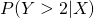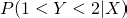# Interval regression with heteroskedastic errors

Interval regression allows one to fit a linear model of an outcome on covariates when the outcome is subject to censoring. In Stata an interval regression can be fitted using the intreg command. Each outcome value is either observed exactly, is interval censored (we know it lies in a certain range), left censored (we only know the outcome is less than some value), or right censored (we only know the outcome is greater than some value). In Stata’s implementation the robust option is available, which with regular linear regression can be used when the residual variance is not constant. Using robust option doesn’t change the parameter estimates, but the standard errors (SEs) are calculated using the sandwich variance estimator. In this post I’ll briefly look at the rationale for using robust with interval regression, and highlight the fact that if the residual variances are not constant, unlike for regular linear regression, the interval regression estimates are biased.

Robust sandwich SEs for regular linear regression
As I’ve discussed in previous posts, in regular linear regression, if the residual variance is not constant, the regression parameter estimates remain unbiased, but the SEs do not. One route to handling the bias in the SEs is to use Huber/White sandwich SEs. To illustrate this, we generate some simple (X,Y) data, where Y follows a linear regression given X, but with residual variance that is a function of X, thus violating the constant variance assumption:

```clear
set seed 1234
set obs 100000
gen x=3*runiform()
gen res_sd=exp(x)
gen y=x+res_sd*rnormal()
```

If we then run the linear regression, first without the robust option, and then with, we obtain:

```. reg y x

Source |       SS       df       MS              Number of obs =  100000
-------------+------------------------------           F(  1, 99998) = 1188.55
Model |  78897.8401     1  78897.8401           Prob > F      =  0.0000
Residual |  6638041.59 99998  66.3817435           R-squared     =  0.0117
Total |  6716939.43 99999   67.170066           Root MSE      =  8.1475

------------------------------------------------------------------------------
y |      Coef.   Std. Err.      t    P>|t|     [95% Conf. Interval]
-------------+----------------------------------------------------------------
x |   1.027518   .0298045    34.48   0.000     .9691014    1.085934
_cons |  -.0177612   .0514732    -0.35   0.730    -.1186481    .0831258
------------------------------------------------------------------------------

. reg y x, robust

Linear regression                                      Number of obs =  100000
F(  1, 99998) =  713.39
Prob > F      =  0.0000
R-squared     =  0.0117
Root MSE      =  8.1475

------------------------------------------------------------------------------
|               Robust
y |      Coef.   Std. Err.      t    P>|t|     [95% Conf. Interval]
-------------+----------------------------------------------------------------
x |   1.027518   .0384705    26.71   0.000     .9521162    1.102919
_cons |  -.0177612   .0359651    -0.49   0.621    -.0882524    .0527301
------------------------------------------------------------------------------
```

The true regression coefficient between Y and X used to generate the data is 1, and we see that out estimates are unbiased (close to 1), despite the non-constant residual variance. The difference between the model based and robust SEs is due to the fact the robust SEs relax the constant variance assumption, which for this (large) dataset is violated.

Interval regression
As outlined above, interval regression allows us to handle the situation where for some records the outcome’s value is not exactly observed, but is subject to interval, left or right censoring. Interval regression accommodates this by including the likelihood contribution from a censored record by calculating the corresponding probability that the outcome value lies in the known range. For example, if we only know that an outcome value is greater than 2 for a particular record/individual, Stata calculates the likelihood contribution corresponding to. If we only know that Y lies between 1 and 2, the contribution is.

Stata’s intreg command also allows the robust option to be used, which gives us a valid estimate of sampling variance for the parameter estimates. One might reasonably think that doing this would allow us to obtain valid inferences even when the errors have non-constant variance. However, unlike in the case of regular linear regression, it turns out that the parameter estimates are biased in general when the errors have non-constant variance. This is because the handling of censored observations in the likelihood calculation relies on the distributional assumption of normality and constant variance of the residual errors. To empirically demonstrate this, we can take our simulated dataset, censor some of the outcome values, and use intreg to fit the regression model:

```. gen depvar1=y

. replace depvar1=2 if y>2

. gen depvar2=y

. replace depvar2=. if y>2
(38110 real changes made, 38110 to missing)

.
. intreg depvar1 depvar2 x

Fitting constant-only model:

Iteration 0:   log likelihood = -247972.92
Iteration 1:   log likelihood = -236859.06
Iteration 2:   log likelihood = -236635.97
Iteration 3:   log likelihood = -236635.67
Iteration 4:   log likelihood = -236635.67

Fitting full model:

Iteration 0:   log likelihood = -248343.77
Iteration 1:   log likelihood = -236509.35
Iteration 2:   log likelihood = -236241.11
Iteration 3:   log likelihood = -236240.65
Iteration 4:   log likelihood = -236240.65

Interval regression                               Number of obs   =     100000
LR chi2(1)      =     790.03
Log likelihood = -236240.65                       Prob > chi2     =     0.0000

------------------------------------------------------------------------------
|      Coef.   Std. Err.      z    P>|z|     [95% Conf. Interval]
-------------+----------------------------------------------------------------
x |  -.7679952   .0268782   -28.57   0.000    -.8206754    -.715315
_cons |   2.222817   .0452328    49.14   0.000     2.134162    2.311471
-------------+----------------------------------------------------------------
/lnsigma |   1.929401   .0030675   628.97   0.000     1.923389    1.935414
-------------+----------------------------------------------------------------
sigma |   6.885387   .0211212                      6.844114    6.926908
------------------------------------------------------------------------------

Observation summary:         0  left-censored observations
61890     uncensored observations
38110 right-censored observations
0       interval observations
```

The intercept and coefficient of X are now biased (estimates of 2.22 and -0.77) from their true values of 0 and 1 respectively – a consequence of the non-constant residual variance. Thus whereas for standard linear regression non-constant residual variance does not bias estimates, for interval regression it does. We could now go on to use robust standard errors:

```. intreg depvar1 depvar2 x, robust

Fitting constant-only model:

Iteration 0:   log pseudolikelihood = -247972.92
Iteration 1:   log pseudolikelihood = -236859.06
Iteration 2:   log pseudolikelihood = -236635.97
Iteration 3:   log pseudolikelihood = -236635.67
Iteration 4:   log pseudolikelihood = -236635.67

Fitting full model:

Iteration 0:   log pseudolikelihood = -248343.77
Iteration 1:   log pseudolikelihood = -236509.35
Iteration 2:   log pseudolikelihood = -236241.11
Iteration 3:   log pseudolikelihood = -236240.65
Iteration 4:   log pseudolikelihood = -236240.65

Interval regression                               Number of obs   =     100000
Wald chi2(1)    =     751.14
Log pseudolikelihood = -236240.65                 Prob > chi2     =     0.0000

------------------------------------------------------------------------------
|               Robust
|      Coef.   Std. Err.      z    P>|z|     [95% Conf. Interval]
-------------+----------------------------------------------------------------
x |  -.7679952    .028022   -27.41   0.000    -.8229173   -.7130731
_cons |   2.222817     .03622    61.37   0.000     2.151827    2.293807
-------------+----------------------------------------------------------------
/lnsigma |   1.929401   .0062859   306.94   0.000     1.917081    1.941722
-------------+----------------------------------------------------------------
sigma |   6.885387   .0432812                      6.801078    6.970741
------------------------------------------------------------------------------

Observation summary:         0  left-censored observations
61890     uncensored observations
38110 right-censored observations
0       interval observations
```

Using robust here does change the SE for the intercept/constant somewhat, but the problem is that using robust doesn’t affect the parameter estimates, which are still biased.

Conclusion
To conclude, if outcomes are subject to censoring, and we think the outcome regression has non-constant residual variance, or non-normal errors, our estimates based on interval regression (assuming normally distributed constant variance errors) will in general be biased. This is not a deficiency of interval regression per se, but simply is a reflection that to handle censoring, the distributional assumption on the errors is much more important than in standard linear regression.

### 2 thoughts on “Interval regression with heteroskedastic errors”

1.I found this very interesting. I’m just wondering whether there is a typo or error in the last sentence of the paragraph with heading “Interval Regression” that reads “the contribution is P(1”?

•